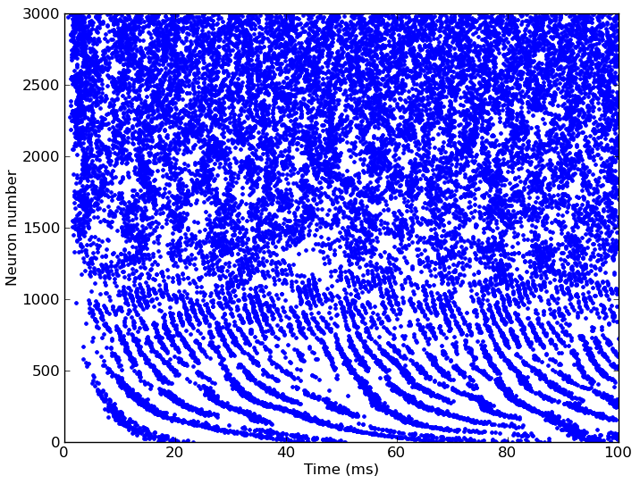# Connecting with Brian¶

To create spiking neuron models based on filter chains, you use the FilterbankGroup class. This acts exactly like a standard Brian NeuronGroup except that you give a source filterbank and choose a state variable in the target equations for the output of the filterbank. A simple auditory nerve fibre model would take the inner hair cell model from earlier, and feed it into a noisy leaky integrate-and-fire model as follows:

# Inner hair cell model as before
cfmin, cfmax, cfN = 20*Hz, 20*kHz, 3000
cf = erbspace(cfmin, cfmax, cfN)
sound = Sound.whitenoise(100*ms)
gfb = Gammatone(sound, cf)
ihc = FunctionFilterbank(gfb, lambda x: 3*clip(x, 0, Inf)**(1.0/3.0))
# Leaky integrate-and-fire model with noise and refractoriness
eqs = '''
dv/dt = (I-v)/(1*ms)+0.2*xi*(2/(1*ms))**.5 : 1 (unless refractory)
I : 1
'''
G = FilterbankGroup(ihc, 'I', eqs, reset='v=0', threshold='v>1', refractory=5*ms)
# Run, and raster plot of the spikes
M = SpikeMonitor(G)
run(sound.duration)
plot(M.t/ms, M.i, '.')
show()


And here’s the output: